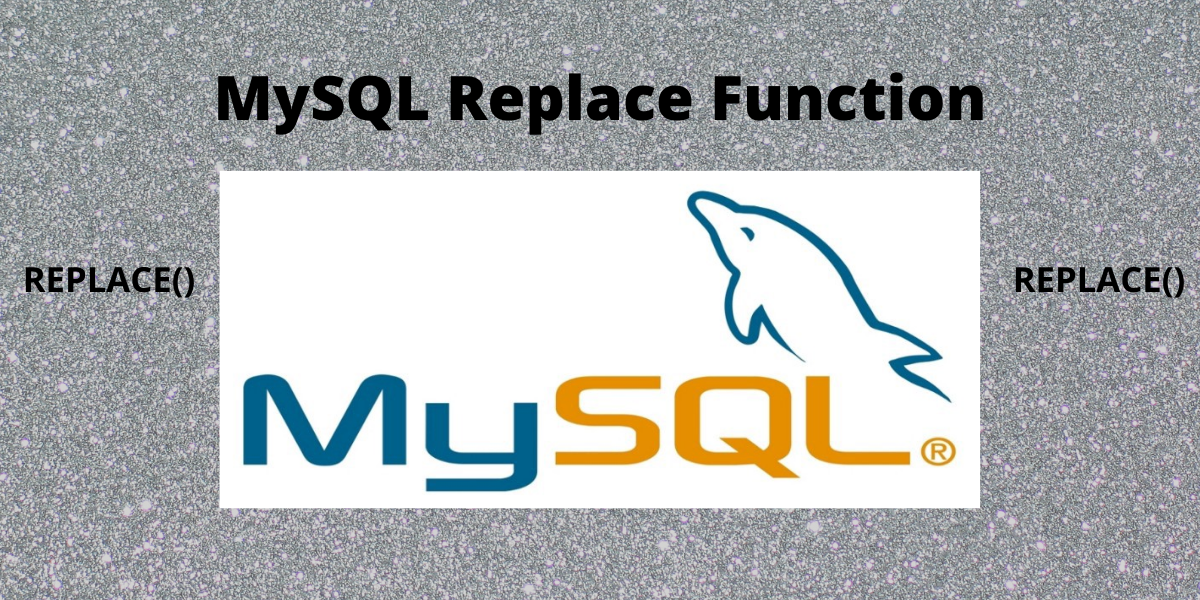# MySQL Replace – Addon UsesIn this tutorial, we will study REPLACE() function in MySQL to insert and update data in tables.

Also read: MySQL REPLACE() – Edit Strings and Replace Values in MySQL

## Introduction to MySQL REPLACE()

In MySQL, Replace statement is used to replace the data in the tables. The process is executed by inserting a new row in the table if there are duplicate key errors. If the insertion fails due to a duplicate key error then delete that row and again insert a new row in the table again.

The MySQL REPLACE() string function and REPLACE() are both different topics and not the same. When using the REPLACE() statement, the user needs to have both Insert and Delete privileges for the table.

## To Insert a new row using MySQL REPLACE()

The syntax for inserting a new row in a table is-

```Replace [INTO] table_name(column_list)
Values(value_list);
```

To illustrate this example, first, we will create a table named t1 and insert rows into it. The syntax for the following is-

```Create Table t1 (
Id int Auto_increment Primary Key,
Name varchar(100),
marks int Not Null
);

Insert into t1(Name, marks)
Values('Joe', 55),
('Jane', 79),
('Preeti', 88);

Select * from t1;
```

Output-

Now we will use REPLACE() statement to change the marks of Jane. The code for the following-

```Replace INTO t1(Id,marks)
Values(2,90);
```

Output-

## To update a row using MySQL REPLACE()

The syntax for updating data in the table is-

```Replace INTO table
SET column1 = value1,
column2 = value2;
```

Let us update the table. The code for the following is-

``` Replace into t1
set Id = 5,
Name = 'Rue',
marks = 45;

Select * from t1;
```

Output-

## To insert data from Select statement using MySQL REPLACE()

The syntax for the following is-

```Replace Into table_1(column_list)
Select column_list
From table_2
WHERE where_condition;
```

Let us make changes in table t1. The code for the following is-

```Replace Into
t1(Name, marks)
Select
Name, marks
From t1
Where Id = 2;
```

Output-